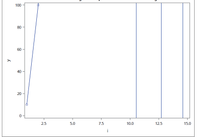## PROC SGPLOT and ODS PDF errors with extremely large values

I'm having trouble graphing data with extremely large values.

Here is an example that reproduces the problem:

``````data dummy;
do i = 1 to 15;
y = 10**i;
output;
end;
run;

ods pdf file="problem.pdf";
proc sgplot data=dummy;
series x=i y=y / markers;
yaxis min=0 max=100;
run;
ods pdf close;``````

Everything looks as expected in results viewer: I see the first two points and the rest is blank.

But the PDF does not look the same. Here is a screenshot of the graph:There are 3 vertical lines on the right that should not be there.

What's strange is that when I zoom out (e.g. yaxis min=0 max=100000), the location and number of these lines change. And if I do not restrict the zoom at all, they disappear.

I think the large numbers are somehow interacting with the upper yaxis max value.

Any ideas how I can get the pdf output to match the desired output in the results viewer?

System Info: SAS 9.4 TS Level 1M7 on Windows.

Thanks!

1 ACCEPTED SOLUTION

Accepted SolutionsDanH_sas
SAS Super FREQ

## Re: PROC SGPLOT and ODS PDF errors with extremely large values

This issue seems to be addressed in SAS 9.4 TS level M8. As a workaround, you might be able to filter out the extreme values using a WHERE clause on the SGPLOT procedure.

3 REPLIES 3

## Re: PROC SGPLOT and ODS PDF errors with extremely large values

If you are willing to accept different XAXIS labels this seems to "work"

```proc sgplot data=dummy ;
where y<10000;
series x=i y=y / markers;
yaxis min=0 max=100  ;
xaxis values=(0 to 15 by 1);
run;
```

But this approach may not work with your "real" data assuming this is a simplification of something else. I might ask why the extremely small limit on the min/max range given the range of the actual Y values.

If you only have a single or small number of values outside the range you want to display perhaps min and max filter correctly. But if most of the actual values are that large perhaps you want to look at a Type=LOG scale for the Y axis.

@AndrewB wrote:

I'm having trouble graphing data with extremely large values.

Here is an example that reproduces the problem:

``````data dummy;
do i = 1 to 15;
y = 10**i;
output;
end;
run;

ods pdf file="problem.pdf";
proc sgplot data=dummy;
series x=i y=y / markers;
yaxis min=0 max=100;
run;
ods pdf close;``````

Everything looks as expected in results viewer: I see the first two points and the rest is blank.

But the PDF does not look the same. Here is a screenshot of the graph:There are 3 vertical lines on the right that should not be there.

What's strange is that when I zoom out (e.g. yaxis min=0 max=100000), the location and number of these lines change. And if I do not restrict the zoom at all, they disappear.

I think the large numbers are somehow interacting with the upper yaxis max value.

Any ideas how I can get the pdf output to match the desired output in the results viewer?

System Info: SAS 9.4 TS Level 1M7 on Windows.

Thanks!

## Re: PROC SGPLOT and ODS PDF errors with extremely large values

Thanks -- unfortunately the x-axis trick didn't work on my real data. As for the motivation for the tight zoom of these graphs, their purpose is to look at the "usual" pattern of simulation replicates, but a few replicates do not converge and go off to infinity. I want that to be shown as the line exiting the graph (like the only line in my example), but I am not interested in its behavior after it has blown up.DanH_sas
SAS Super FREQ

## Re: PROC SGPLOT and ODS PDF errors with extremely large values

This issue seems to be addressed in SAS 9.4 TS level M8. As a workaround, you might be able to filter out the extreme values using a WHERE clause on the SGPLOT procedure.

Discussion stats
• 3 replies
• 213 views
• 1 like
• 3 in conversation